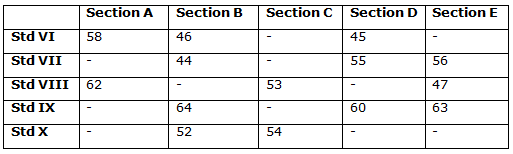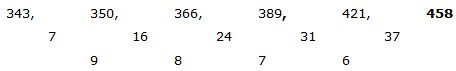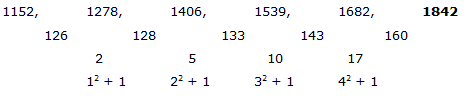# IBPS SO / NIACL AO Prelims – Quantitative Aptitude Questions Day- 54

Dear Readers, Bank Exam Race for the Year 2018 is already started, To enrich your preparation here we have providing new series of Practice Questions on Quantitative Aptitude – Section. Candidates those who are preparing for IBPS SO / NIACL AO Prelims 2018 Exams can practice these questions daily and make your preparation effective.

[WpProQuiz 4616]

Directions (Q. 1 – 5) Study the following information carefully and answer the given questions:

The following table shows the total number of students studying in different sections of different standards. Some values are missing here.1) Find the difference between the total students in Section A and C together in Std VI to that of total students in Section B and E together in std VIII, if the total students in Section C in std VI is 2 more than the average students in Section D in Std VI and VII together and the total students in Section B in std VIII is 6 more than the total students in Section A in std VIII?

a) 5

b) 15

c) 25

d) 30

e) None of these

2) Find the total number of students in Std VII of all the given sections together, if the ratio between the total number of students in section A to that of Section C in std VII is 5 : 6 and the total number of students in section E in Std IX is 15 more than the total number of students in section C of std VII?

a) 243

b) 234

c) 246

d) 218

e) None of these

3) Total number of students in Section E of std VIII and IX together is what percentage of total number of students in Section B of Std VI and IX together?

a) 125 %

b) 130 %

c) 140 %

d) 100 %

e) None of these

4) If the average number of students in Section D of all the given Std together is 55 and the total number of students in Section D in Std X is one more than the total number of students in Section D in Std VIII, then find the total number of students in Section D of std X?

a) 62

b) 58

c) 60

d) 64

e) None of these

5) Find the ratio between the total number of students in Section A of std VI and VIII together to that of total number of students in Section B of std VI and VII together?

a) 5: 4

b) 7: 5

c) 4: 3

d) 8: 3

e) None of these

Directions (Q. 6 – 10): Find the wrong term in the following number series

6) 343, 350, 366, 390, 421, 457

a) 343

b) 350

c) 457

d) 366

e) 390

7) 12, 26, 81, 327, 1645, 9876

a) 327

b) 26

c) 81

d) 1645

e) 9876

8) 5, 10, 71, 551, 4751

a) 71

b) 551

c) 4751

d) 10

e) 5

9) 1152, 1278, 1406, 1539, 1682, 1840

a) 1278

b) 1840

c) 1539

d) 1682

e) 1406

10) 8, 57, 392, 2353, 11761

a) 392

b) 8

c) 57

d) 2353

e) 11761

Direction (1-5) :

The total students in Section C in std VI

= > The average students in Section D in Std VI and VII together + 2

= > [(45 + 55)/2] + 2 = 52

The total students in Section A and C together in Std VI

= > 58 + 52 = 110

The total students in Section B in std VIII

= > 6 + The total students in Section A in std VIII

= > 6 + 62 = 68

The total students in Section B and E together in Std VIII

= > 68 + 47 = 115

Required difference = 115 – 110 = 5

The ratio between the total number of students in section A to that of Section C in std VII = 5 : 6 (5x, 6x)

The total number of students in section E in Std IX = 15 + The total number of students in section C of std VII

The total number of students in section C of std VII

= > 63 – 15 = 48

The total number of students in section A in std VII

= > (48/6)*5 = 40

The total number of students in Std VII of all the given sections together

= > 40 + 44 + 48 + 55 + 56 = 243

Total number of students in Section E of std VIII and IX together

= > 47 + 63 = 110

Total number of students in Section B of Std VI and IX together

= > 46 + 64 = 110

Required % = (110/110)*100 = 100 %

The average number of students in Section D of all the given Std together = 55

Total number of students in Section D of all the given Std together

= > 55*5 = 275

VI + VII + VIII + IX + X = 275

45 + 55 + VIII + 60 + X = 275

X + VIII = 115 → (1)

The total number of students in Section D in Std X = 1 + The total number of students in Section D in Std VIII

X – VIII = 1 →(2)

By solving the equation (1) and (2), we get,

X = 58, VIII = 57

The total number of students in Section D in Std X = 58

The total number of students in Section A of std VI and VIII together

= > 58 + 62 = 120

The total number of students in Section B of std VI and VII together

= > 46 + 44 = 90

Required ratio = 120: 90 = 4: 3

Direction (6-10) :

The correct series is,The difference of difference is, 9, 8, 7, 6,….

The wrong term is, 457

The correct series is,

12, 26, 81, 328, 1645, 9876

The pattern is, *2 + 2, *3 + 3, *4 + 4, *5 + 5, *6 + 6,…

The wrong term is, 327

The correct series is,

5, 11, 71, 551, 4751

The pattern is, *2 + 13, *4 + 33, *6 + 53, *8 + 73,…

The wrong term is, 10

The correct series is,The difference of difference is, 12 + 1, 22 + 2, 32 + 1, 42 + 1,…

The wrong term is, 1840

The correct series is,

8, 57, 393, 2353, 11761

The pattern is, *8 – 7, *7 – 6, *6 – 5, *5 – 4,…

The wrong term is, 392

Daily Practice Test Schedule | Good Luck

 Topic Daily Publishing Time Daily News Papers & Editorials 8.00 AM Current Affairs Quiz 9.00 AM Current Affairs Quiz (Hindi) 9.30 AM IBPS SO/NIACL AO Prelims – Reasoning 10.00 AM IBPS SO/NIACL AO Prelims – Reasoning (Hindi) 10.30 AM IBPS SO/NIACL AO Prelims – Quantitative Aptitude 11.00 AM IBPS SO/NIACL AO Prelims – Quantitative Aptitude (Hindi) 11.30 AM Vocabulary (Based on The Hindu) 12.00 PM IBPS SO/NIACL AO Prelims – English Language 1.00 PM SSC Practice Questions (Reasoning/Quantitative aptitude) 2.00 PM IBPS Clerk – GK Questions 3.00 PM SSC Practice Questions (English/General Knowledge) 4.00 PM Daily Current Affairs Updates 5.00 PM SBI PO/IBPS Clerk Mains – Reasoning 6.00 PM SBI PO/IBPS Clerk Mains – Quantitative Aptitude 7.00 PM SBI PO/IBPS Clerk Mains – English Language 8.00 PM